# Number unknown

Adela thought the two-digit number, she added it to its ten times and got 407. What number does she think?

Correct result:

a =  37

#### Solution:

a+10a = 407

a+10•a = 407

11a = 407

a = 37

Calculated by our simple equation calculator.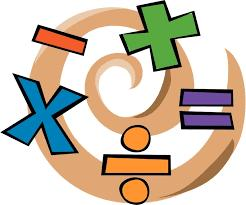We would be very happy if you find an error in the example, spelling mistakes, or inaccuracies, and please send it to us. We thank you!Tips to related online calculators
Do you have a linear equation or system of equations and looking for its solution? Or do you have quadratic equation?

## Next similar math problems:

• Unknown number xyzFind the number that its triple is 24. Solve by equation.
• Mystery numberA mystery number is between 800 and 920. No digits are the same. The difference of the numbers 5. What is the mystery number?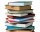Readers borrowed a total of 220 books in the library during the first three days. On the second day, readers borrowed half as many books as the first day and at the same time 20 fewer books than the third day. Depending on the quantity x, express the numb
• I thinkI think a number. When I multiply it by five, and after that I subtract 477, I get the same number as if I multiplied it twice. What number do I think?
• What is missing (1000)What number is to add to get 1000?
• Seven timesWhich number seven times is just as higher as 27, how much is smaller than 29?
• The number 3Ski organizers should print the start numbers from 1 to 45. How many times will they use the number 3 when printing?
• Number of songs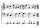Write an expression for the number of songs they need for this show. Evan and Peter have a radio show that has 2 parts. They need 4 fewer than 11 songs in the first part. In the second part, they need 5 fewer than 3 times the number of songs in the first
• Equation with one variableSolve the following equation with one unknown: 5(7s + 5) =130
• Roman numeralsWrite numbers written in Roman numerals as decimal.
• Expression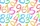If A=2 B=3 evaluate expression A(B+A) and multiply it by A
• SummandOne of the summands is 145. The second is 10 more. Determine the sum of the summands.
• Discount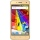The new phone was discounted by 2800kč. After this discount, 5 phones cost 5530 CZK more than 3 phones before the discount. How much did the phone cost before the discount?
• Eq1Solve equation: 4(a-3)=3(2a-5)
• Hotel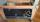The rooms in the mountain hotel are double and triple. Double rooms are 25 and triple are 17 more. How many rooms are there in this hotel?
• Six te 2If 3t-7=5t, then 6t=
• If-then equationIf 5x - 17 = -x + 7, then x =